# CHAPTER 2 2 CONTROL STRUCTURES ITERATION Dr Shady

• Slides: 18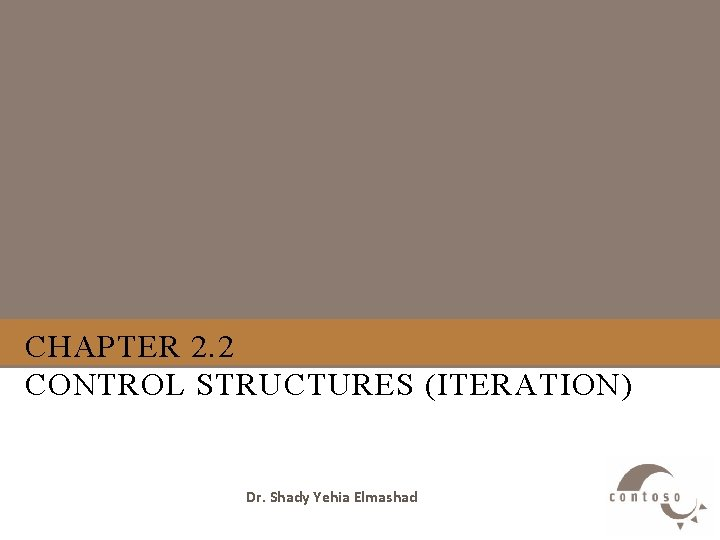Outline 1. 2. 3. 4. 5. 6. C++ Iterative Constructs The for Repetition Structure Examples Using the for Structure The while Repetition Structure Examples Using the while Structure Formulating Algorithms (Counter-Controlled Repetition) 7. Formulating Algorithms with Top-Down, Stepwise Refinement 8. Nested control structures 9. Essentials of Counter-Controlled Repetition 10. The do/while Repetition Structure 11. The break and continue Statements1. C++ Iterative Constructs • There are three constructs: Ø while statement Ø for statement Ø do-while statement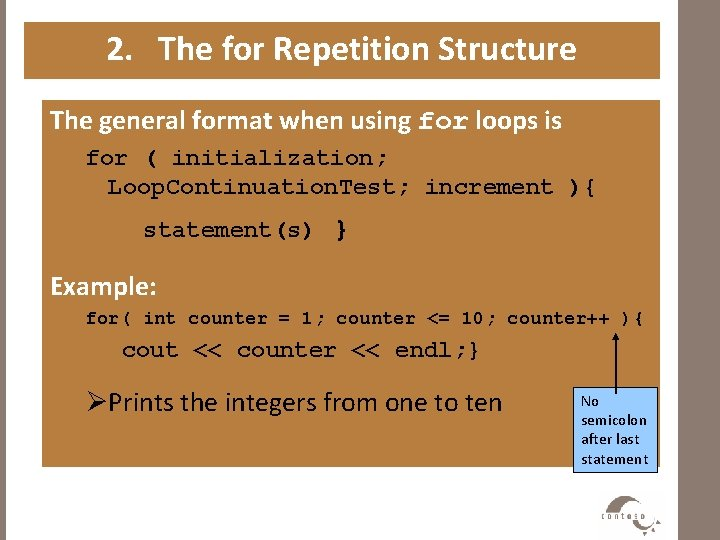2. The for Repetition Structure The general format when using for loops is for ( initialization; Loop. Continuation. Test; increment ){ statement(s) } Example: for( int counter = 1; counter <= 10; counter++ ){ cout << counter << endl; } ØPrints the integers from one to ten No semicolon after last statement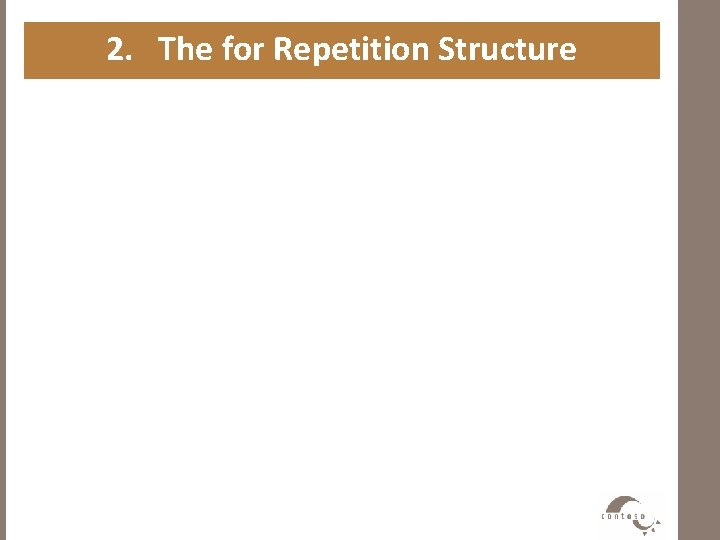2. The for Repetition Structure • Syntax for (For. Init ; For. Expression; Post. Expression) Action • Example for (int i = 0; i < 3; ++i) { cout << "i is " << i << endl; }2. The for Repetition Structure • For loops can usually be rewritten as while loops: initialization; while ( loop. Continuation. Test){ statement increment; } • Initialization and increment as comma-separated lists for (int i = 0, j = 0; j + i <= 10; j++, i++) cout << j + i << endl;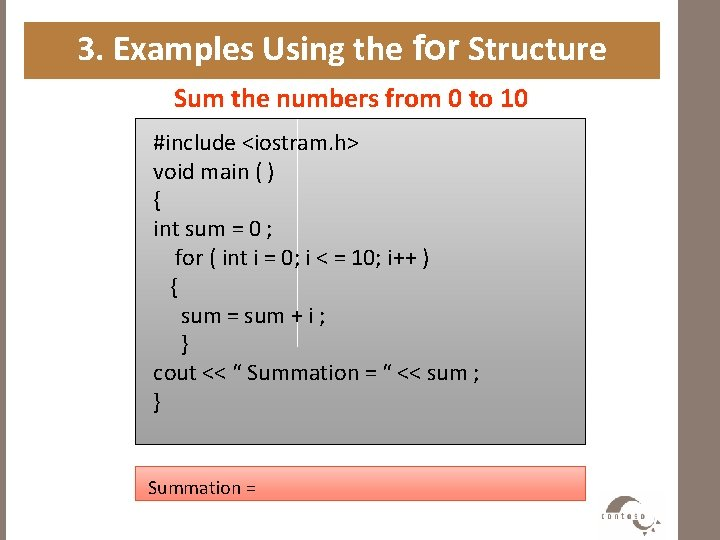3. Examples Using the for Structure Sum the numbers from 0 to 10 #include <iostram. h> void main ( ) { int sum = 0 ; for ( int i = 0; i < = 10; i++ ) { sum = sum + i ; } cout << “ Summation = “ << sum ; } Summation =3. Examples Using the for Structure Sum the even numbers from 0 to 100 #include <iostram. h> void main ( ) { int sum = 0 ; for ( int i = 0; i < = 100; i+=2 ) { sum = sum + i ; } cout << “ Summation = “ << sum ; } Summation =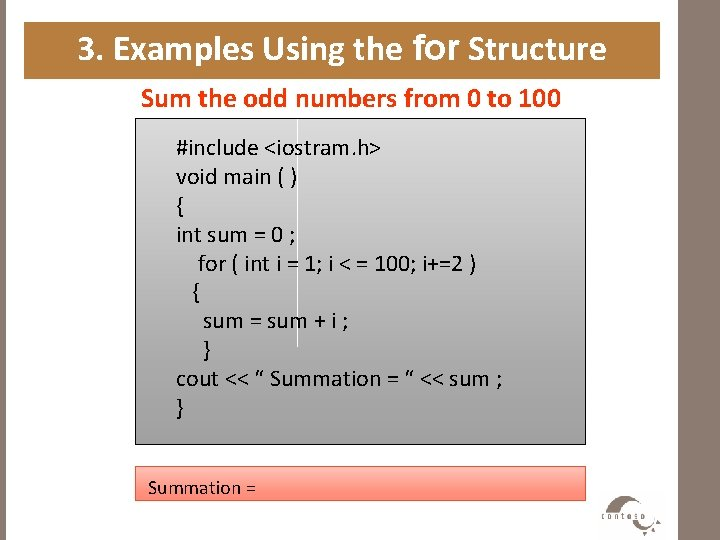3. Examples Using the for Structure Sum the odd numbers from 0 to 100 #include <iostram. h> void main ( ) { int sum = 0 ; for ( int i = 1; i < = 100; i+=2 ) { sum = sum + i ; } cout << “ Summation = “ << sum ; } Summation =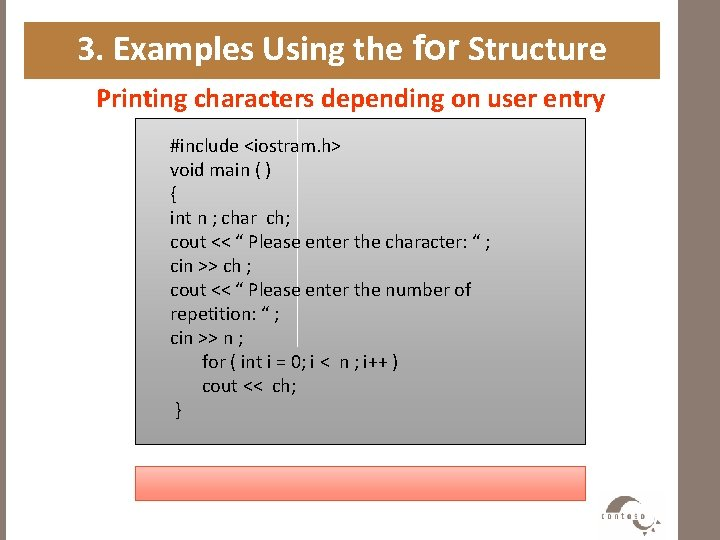3. Examples Using the for Structure Printing characters depending on user entry #include <iostram. h> void main ( ) { int n ; char ch; cout << “ Please enter the character: “ ; cin >> ch ; cout << “ Please enter the number of repetition: “ ; cin >> n ; for ( int i = 0; i < n ; i++ ) cout << ch; }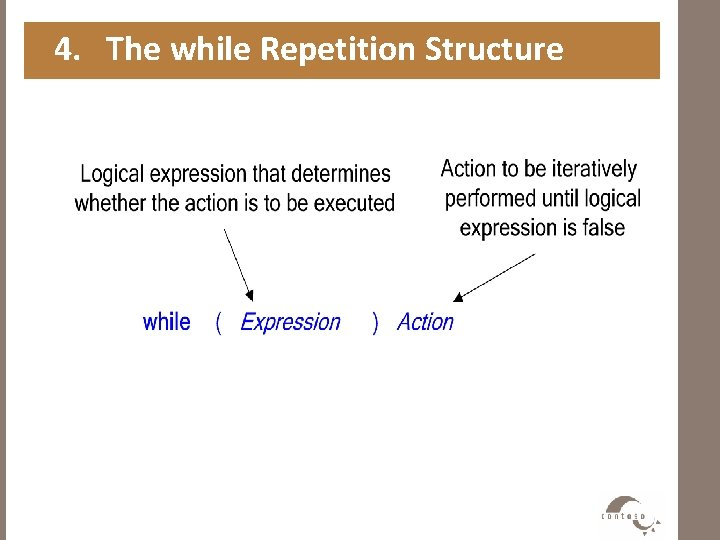4. The while Repetition Structure4. The while Repetition Structure While Semantics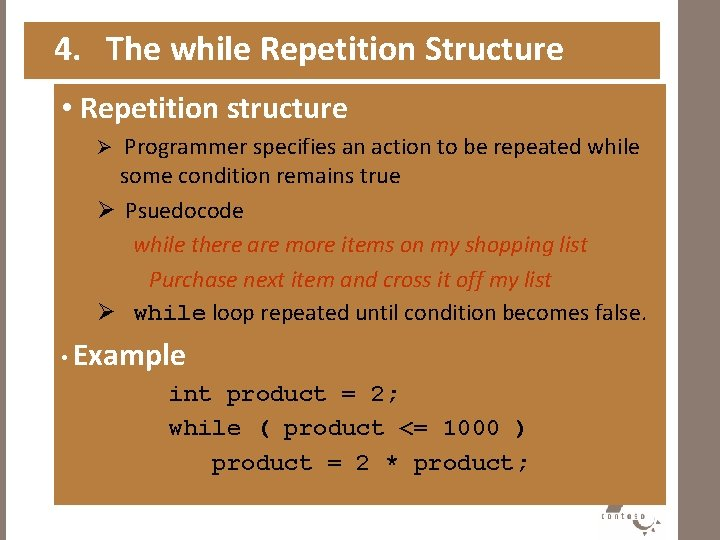4. The while Repetition Structure • Repetition structure Ø Programmer specifies an action to be repeated while some condition remains true Ø Psuedocode while there are more items on my shopping list Purchase next item and cross it off my list Ø while loop repeated until condition becomes false. • Example int product = 2; while ( product <= 1000 ) product = 2 * product;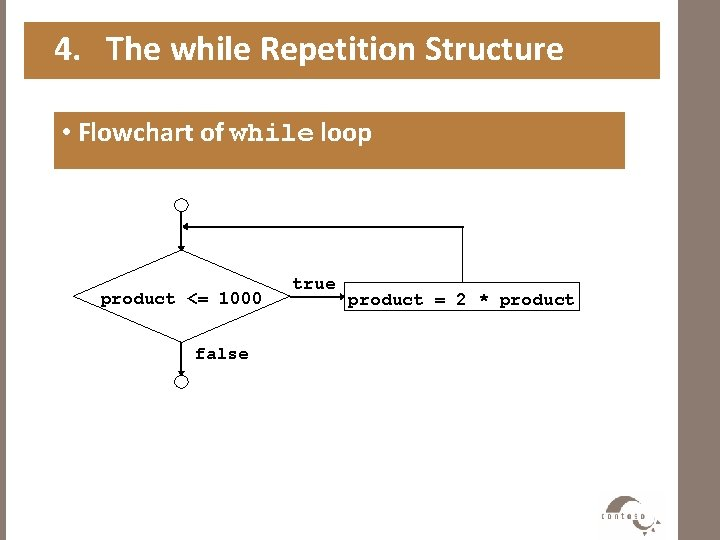4. The while Repetition Structure • Flowchart of while loop product <= 1000 false true product = 2 * product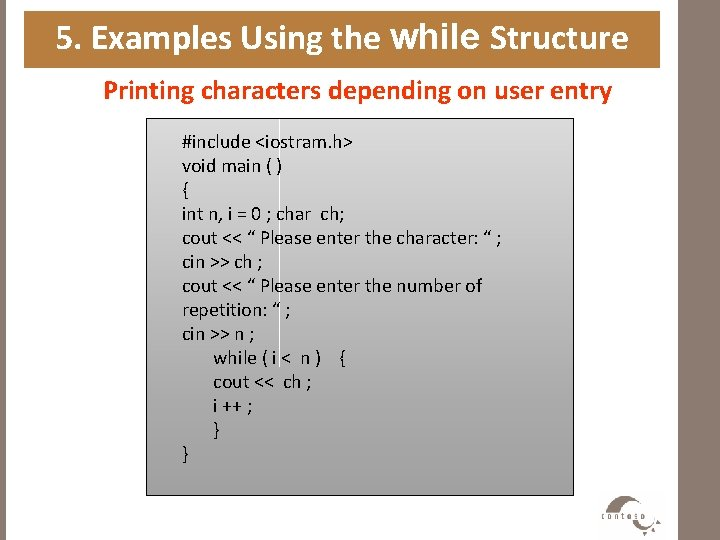5. Examples Using the while Structure Printing characters depending on user entry #include <iostram. h> void main ( ) { int n, i = 0 ; char ch; cout << “ Please enter the character: “ ; cin >> ch ; cout << “ Please enter the number of repetition: “ ; cin >> n ; while ( i < n ) { cout << ch ; i ++ ; } }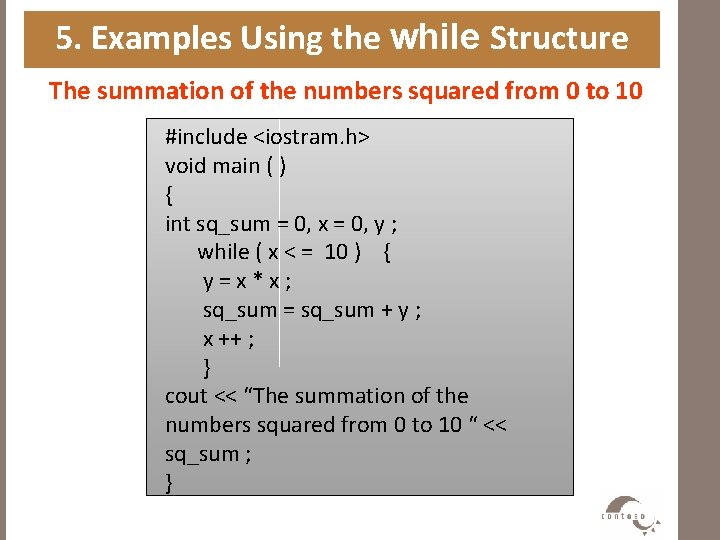5. Examples Using the while Structure The summation of the numbers squared from 0 to 10 #include <iostram. h> void main ( ) { int sq_sum = 0, x = 0, y ; while ( x < = 10 ) { y=x*x; sq_sum = sq_sum + y ; x ++ ; } cout << “The summation of the numbers squared from 0 to 10 “ << sq_sum ; }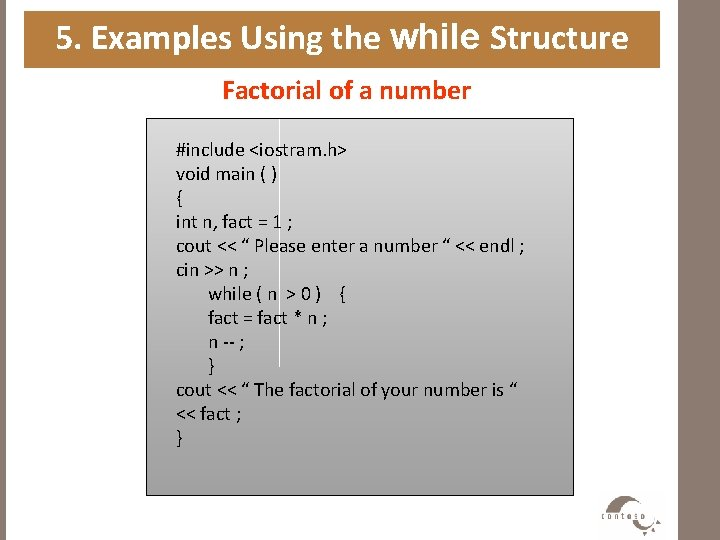5. Examples Using the while Structure Factorial of a number #include <iostram. h> void main ( ) { int n, fact = 1 ; cout << “ Please enter a number “ << endl ; cin >> n ; while ( n > 0 ) { fact = fact * n ; n -- ; } cout << “ The factorial of your number is “ << fact ; }